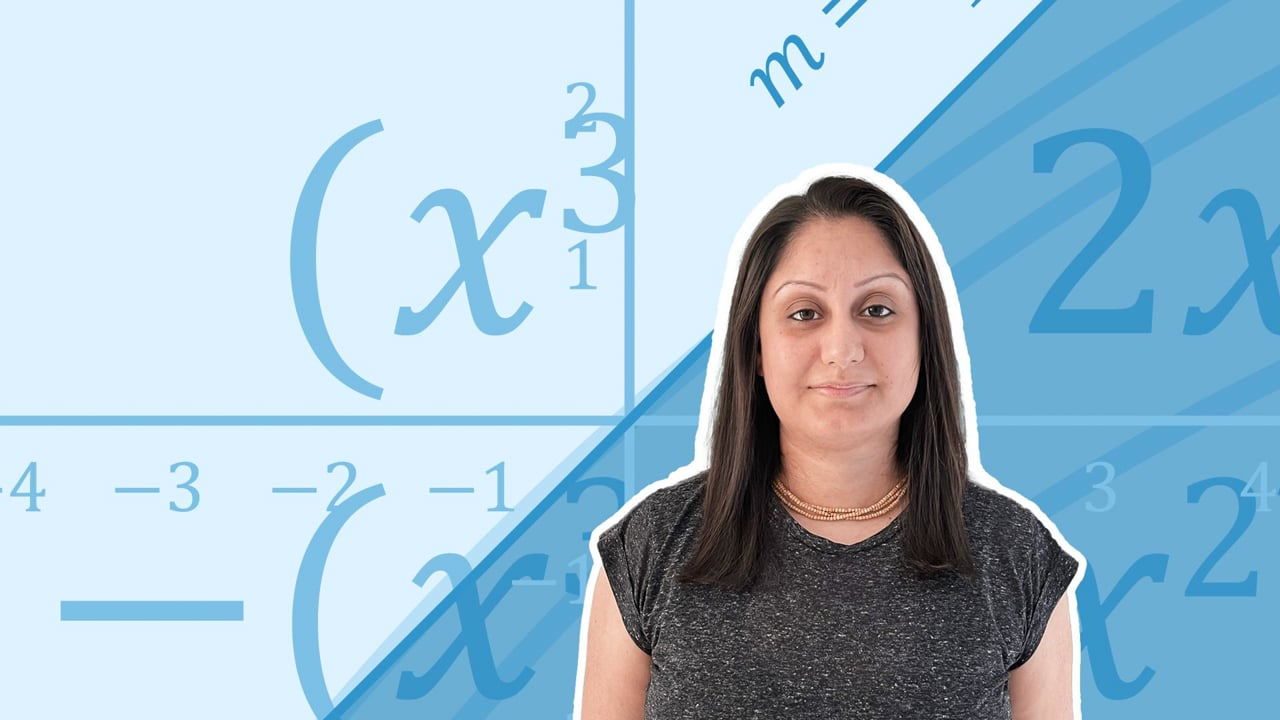Chapter overviewMaths

Types of numbers

Number calculations

Fractions, decimals and percentages

Algebraic manipulation

Formulae and equations

Straight line graphs

Other graphs

Ratio

Proportion

Rates of change

Shapes

Properties of shapes

Measures

Lines and angles

Drawing shapes

Trigonometry

Probability

Statistics

Maths

# Algebraic notation0%

Summary

# Algebraic notation

## In a nutshell

Algebra uses letters called 'variables' to represent unknown quantities. The basic rules for algebraic notation help write terms in a concise way. These terms can then be used in expressions, formulae or equations.

##### Example 1

The letter 'a' could represent an apple. Two apples could be described by $\underline{2a}$.​

## Basic rules

RULE

DESCRIPTION

EXAMPLE

Add the numbers and copy the variable. If there is no number with the variable, it means $1$​. For example, $x$​ means $1x$​​
$x+x=2x$​​

Subtract equal variables

Subtract the numbers and copy the variable.
$7x-5x=2x$​​

Multiply same variables

Multiplying the same variables changes the power. When multiplying add powers, if there is no number with the variable, it means $1$​.
$x \times x = x^2$​​

Multiply different variables

Write the letters without the multiplication sign.
Multiplying different variables will not change their powers.
$x \times y = xy$​​

## Formulae

Formulae help find quantities.

##### Examples
 ​$F=ma$​​ Calculate the force on an obejct from its mass and acceleration. ​$P = 2(l+w)$​​ Calculate the perimeter of a rectangle from its length and width. ​$A=\frac{1}{2} bh$​​ Calculate the area of a triangle from its base and its height.

## Equations

An equation is formed when two expressions equal each other.

##### Examples
 ​$\frac x 2 =5$​​ ​$2x-3=7$​​ ​$x^2 -2x-8=0$​​

FAQs

• Question: What is algebraic notation?

Answer: Algebraic notation involves writing algebraic terms, with a combination of numbers and letters. These terms can then be used further in expressions and equations.

• Question: What are variables?

Answer: Variables are a short hand way to name a quantity. The letter variable can then be used in an expression.

• Question: What is algebra?

Answer: Algebra uses letters called variables to represent unknown quantities.

Theory

Exercises